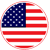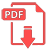# Home | Mathematics | Pre-Algebra

## Roots of a polynomial

We’ll start off this section by defining just what a root or zero of a polynomial is. We say that x=r is a root or zero of a polynomial, P(x), if P(r)=0. In other words, x=r is a root or zero of a polynomial if it is a solution to the equation P(x)=0

.

In the next couple of sections we will need to find all the zeroes for a given polynomial. So, before we get into that we need to get some ideas out of the way regarding zeroes of polynomials that will help us in that process.

The process of finding the zeroes of P(x)
really amount to nothing more than solving the equation P(x)=0 and we already know how to do that for second degree (quadratic) polynomials. So, to help illustrate some of the ideas were going to be looking at let’s get the zeroes of a couple of second degree polynomials.English